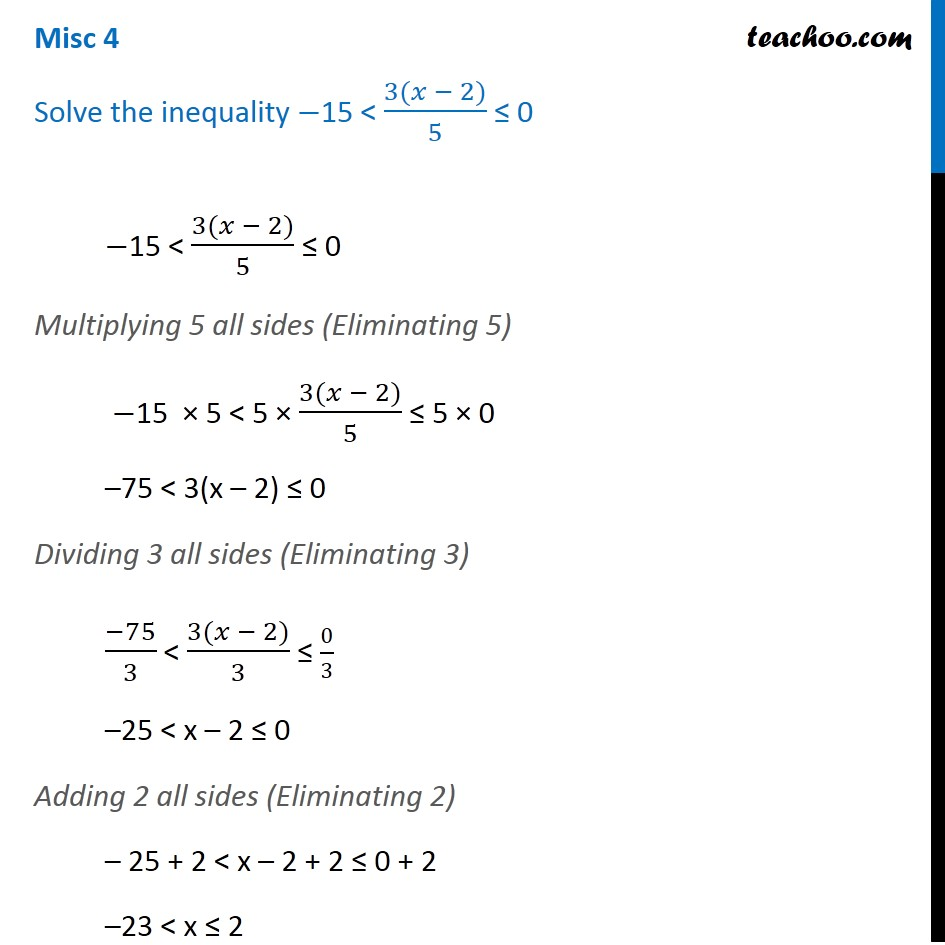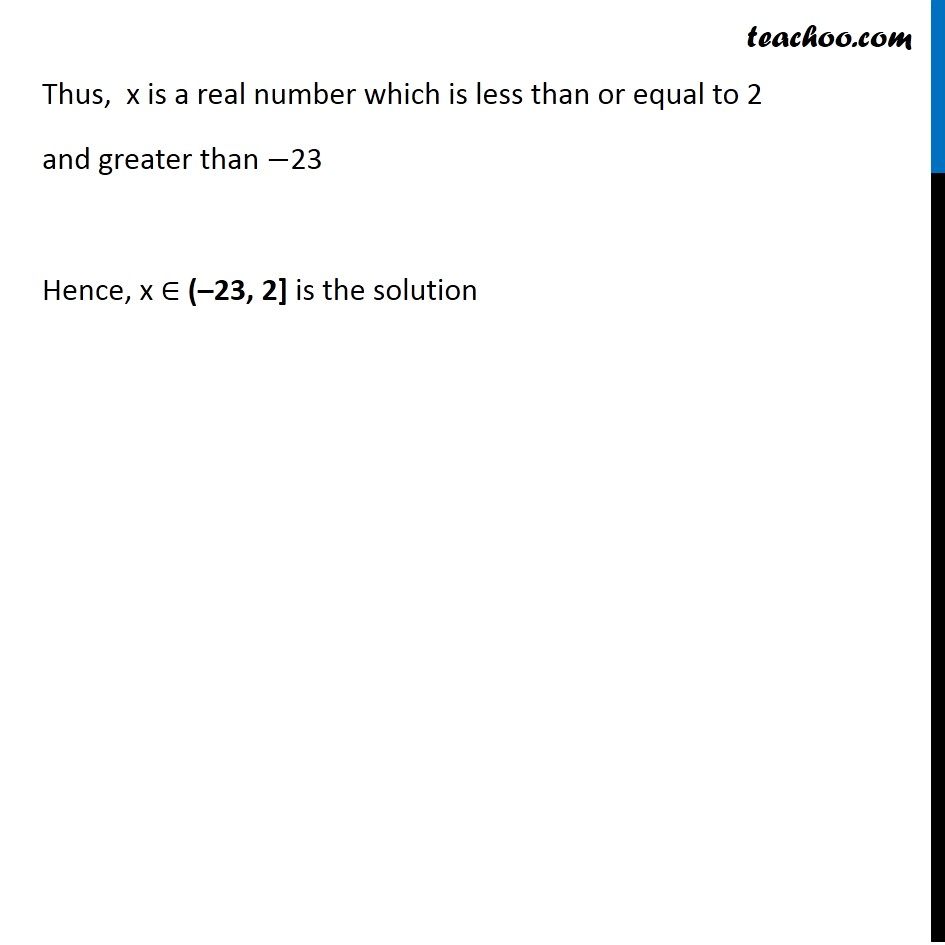Miscellaneous

Chapter 6 Class 11 Linear Inequalities
Serial order wiseGet live Maths 1-on-1 Classs - Class 6 to 12

### Transcript

Misc 4 Solve the inequality −15 < (3(𝑥 − 2))/5 ≤ 0 −15 < (3(𝑥 − 2))/5 ≤ 0 Multiplying 5 all sides (Eliminating 5) −15 × 5 < 5 × (3(𝑥 − 2))/5 ≤ 5 × 0 –75 < 3(x – 2) ≤ 0 Dividing 3 all sides (Eliminating 3) (−75)/3 < (3(𝑥 − 2))/3 ≤ 0/3 –25 < x – 2 ≤ 0 Adding 2 all sides (Eliminating 2) – 25 + 2 < x – 2 + 2 ≤ 0 + 2 –23 < x ≤ 2 Thus, x is a real number which is less than or equal to 2 and greater than −23 Hence, x ∈ (–23, 2] is the solution